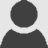# PHP Managed Extensibility Framework – PHPMEF

Edit on GitHubWhile flying sitting in the airplane to the Microsoft Web Developer Summit in Seattle, I was watching some PDC09 sessions on my laptop. During the MEF session, an idea popped up: there is no MEF for PHP! 3500 kilometers after that moment, PHP got its own MEF…

MEF is a .NET library, targeting extensibility of projects. It allows you to declaratively extend your application instead of requiring you to do a lot of plumbing. All this is done with three concepts in mind: export, import and compose. (Glenn, I stole the previous sentence from your blog). “PHPMEF” uses the same concepts in order to provide this extensibility features.

Let’s start with a story… Imagine you are building a Calculator. Yes, shoot me, this is not a sexy sample. Remember I wrote this one a plane with snoring people next to me…The Calculator is built of zero or more ICalculationFunction instances. Think command pattern. Here’s how such an interface can look like:

[code:c#]

interface ICalculationFunction
{
public function execute(\$a, \$b);
}

[/code]

Nothing special yet. Now let’s implement an instance which does sums:

[code:c#]

class Sum implements ICalculationFunction
{
public function execute(\$a, \$b)
{
return \$a + \$b;
}
}

[/code]

Now how would you go about using this in the following Calculator class:

[code:c#]

class Calculator
{
public \$CalculationFunctions;
}

[/code]

Yes, you would do plumbing. Either instantiating the Sum object and adding it into the Calculator constructor, or something similar. Imagine you also have a Division object. And other calculation functions. How would you go about building this in a maintainable and extensible way? Easy: use exports…

### Export

Exports are one of the three fundaments of PHPMEF. Basically, you can specify that you want class X to be “ exported”  for extensibility. Let’s export Sum:

[code:c#]

/**
* @export ICalculationFunction
*/
class Sum implements ICalculationFunction
{
public function execute(\$a, \$b)
{
return \$a + \$b;
}
}

[/code]

Sum is exported as Sum by default, but in this case I want PHPMEF to know that it is also exported as ICalculationFunction. Let’s see why this is in the import part…

### Import

Import is a concept required for PHPMEF to know where to instantiate specific objects. Here’s an example:

[code:c#]

class Calculator
{
/**
* @import ICalculationFunction
*/
public \$SomeFunction;
}

[/code]

In this case, PHPMEF will simply instantiate the first ICalculationFunction instance it can find and assign it to the Calculator::SomeFunction variable. Now think of our first example: we want different calculation functions in our calculator! Here’s how:

[code:c#]

class Calculator
{
/**
*  @import-many ICalculationFunction
*/
public \$CalculationFunctions;
}

[/code]

Easy, no? PHPMEF will ensure that all possible ICalculationFunction instances are added to the Calculator::CalculationFunctions array. Now how is all this being plumbed together? It’s not plumbed! It’s composed!

### Compose

Composing matches all exports and imports in a specific application path. How? Easy! Use the PartInitializer!

[code:c#]

// Create new Calculator instance
\$calculator = new Calculator();

// Satisfy dynamic imports
\$partInitializer = new Microsoft_MEF_PartInitializer();
\$partInitializer->satisfyImports(\$calculator);

[/code]

Easy, no? Ask the PartInitializer to satisfy all imports and you are done!

The above sample was used to demonstrate what PHPMEF is all about. I’m sure you can imagine more complex scenarios. Here are some other possibilities…

### Single instance exports

By default, PHPMEF instantiates a new object every time an import has to be satisfied. However, imagine you want our Sum class to be re-used. You want PHPMEF to assign the same instance over and over again, no matter where and how much it is being imported. Again, no plumbing. Just add a declarative comment:

[code:c#]

/**
* @export ICalculationFunction
*/
class Sum implements ICalculationFunction
{
public function execute(\$a, \$b)
{
return \$a + \$b;
}
}

[/code]

Imagine you want to work with interfaces like mentioned above, but want to use a specific implementation that has certain metadata defined. Again: easy and no plumbing!

My calculator might look like the following:

[code:c#]

class Calculator
{
/**
*  @import-many ICalculationFunction
*/
public \$CalculationFunctions;

/**
*  @import ICalculationFunction
*/
public \$SomethingThatCanDoSums;
}

[/code]

Calculator::SomeThingThatCanDoSums is now constrained: I only want to import something that has the metadata “CanDoSums” attached. Here’s how to create such an export:

[code:c#]

/**
* @export ICalculationFunction
*/
class Sum implements ICalculationFunction
{
public function execute(\$a, \$b)
{
return \$a + \$b;
}
}

[/code]

Here’s an answer to a question you may have: yes, multiple metadata definitions are possible and will be used to determine if an export matches an import.

One small note left: you can also ask the PartInitializer for the metadata defined on a class.

[code:c#]

// Create new Calculator instance
\$calculator = new Calculator();

// Satisfy dynamic imports
\$partInitializer = new Microsoft_MEF_PartInitializer();
\$partInitializer->satisfyImports(\$calculator);

[/code]

## Can I get the source?

No, not yet. For a number of reasons. I first want to make this thing a bit more stable, as well as deciding if all MEF features should be ported. Also, I’m looking for an appropriate name/library to put this in. You may have noticed the Microsoft_* naming, a small hint to the Interop team in incorporating this as another Microsoft library in the PHP world. Yes Vijay, talking to you :-)

This is an imported post. It was imported from my old blog using an automated tool and may contain formatting errors and/or broken images.

Tags:

Updated: